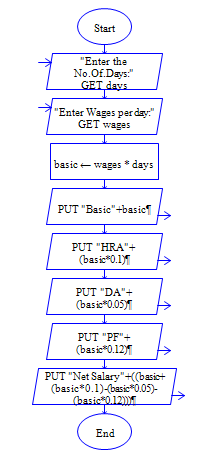# Calculate Salary of Employee in Python

Python program to get employee wages and number of days worked from user and find Basic Pay, DA, HRA, PF and Net Pay.

(Note HRA, DA and PF are 10%,5%and 12% of basicpay respectively.)

Sample Input 1:

300 30

Sample Output 1:

Basic Pay:3000 DA: 150 HRA:300 PF:360 Net Pay: 3090

#### Flow Chart DesignStrongly recommended to Solve it on your own, Don't directly go to the solution given below.

#### Program or Solution

``` days=float(input("Enter No Days Present:")) wages=float(input("Enter wages per Day:")) basic=wages*days; HRA=basic*0.1; DA=basic*0.05; PF=basic*0.12; netsalary=basic+HRA+DA-PF; print("\nBasic:%lf \nHRA:%lf \nDA:%lf \nPF:%lf \nNet Salary:%lf" %(basic,HRA,DA,PF,netsalary)); ```

#### Program Explanation

get wages and number of days present of an employee using input() method.

calculate basic pay, DA, HRA , PF Net Pay using formula